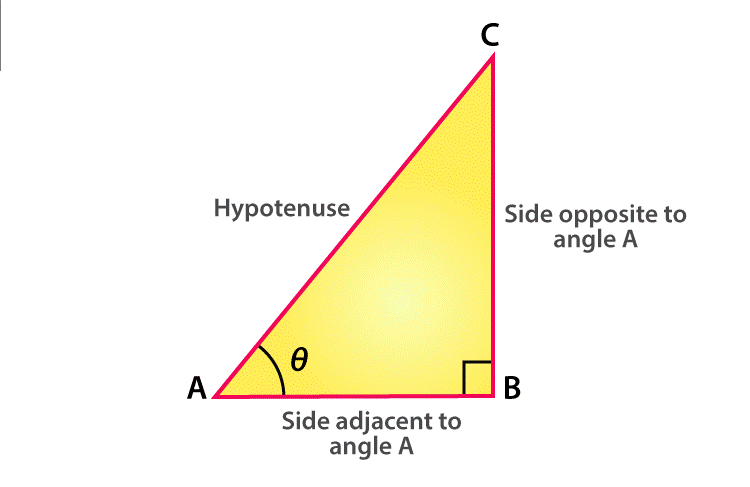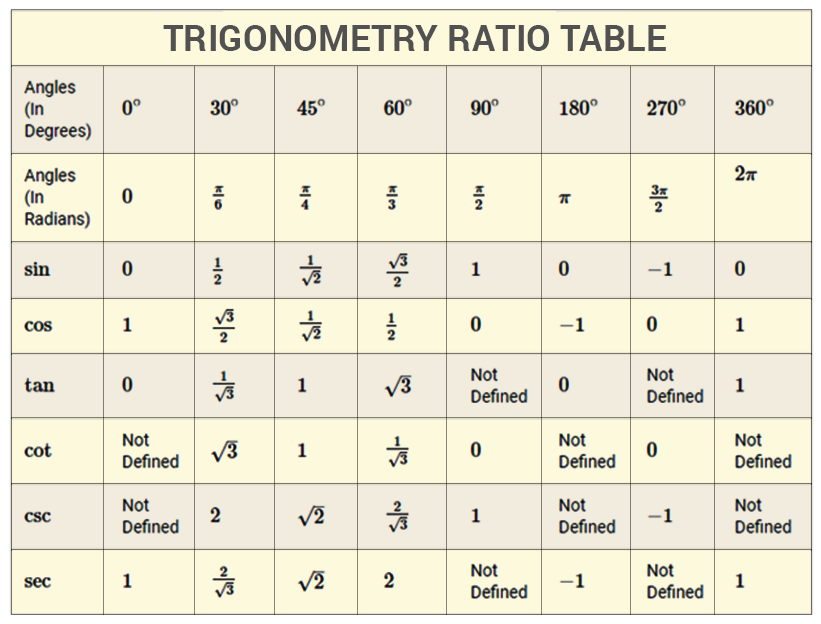# Tan 0

The word ‘ Trigonometry ’ is derived from the Greek word and the subject is developed to solve geometric problems involving triangles. It is used to measure the sides of a triangle. An angle is a measure of rotation of a given ray about its initial point and the original ray is called the initial side and the final position of a ray after the rotation of the original ray is called the terminal side. If the rotation of a ray is in an anticlockwise direction, then the angle is a positive angle and if the rotation of a ray in a clockwise direction, then the angle is a negative angle. An angle is said to be positive if the rotation is in anti clockwise direction and if the rotation is in a clockwise direction, then the angle is negative. The two types of conventions used for measuring angles are

• Degree Measure

In degree measurement, consider a unit circle where the angle is said to have a measure of degree and it is denoted by the symbol ‘1° ‘. Each degree(1°) is divided into 60 minutes (also denoted as 60’ ) and each minute (1’) is subdivided into 60 seconds and it is denoted by 60”. Another unit of measurement of an angle is radian. An angle is said to have a measure of 1 radian in which angle subtended at the centre by an arc length of 1 unit in a unit circle. Generally, trigonometric ratios are represented for the acute angle as the ratio of the sides of a right angle triangle.

## Tan 0 Degree Value

In a right angled triangle, the opposite side of the right angle is called the hypotenuse side, the side opposite the angle of interest is called the opposite side and the remaining side is called the adjacent side where it forms a side of both the right angle and the angle of interest.

The tangent function of an angle is equal to the length of the opposite side divided by the length of the adjacent side.Tan θ = Opposite side/ Adjacent Side

By representing the tangent function in terms of sin and cos function, it is given by

Tan θ = Sin θ / Cos θ

## Deriving the Value of Tan Degrees

To find the value of tan 0 degree, use sine function and cosine function. Because tan function is the ratio of sine function and cos function.

We can easily learn the values of tangent degrees with the help of sine functions and cosine functions. Just knowing the value of sine functions, we will find the values of cos and tan functions. There is an easy way to remember Some other tangent values of like tan 30, tan 60 degrees..

Sin 0° = $\sqrt{\frac{0}{4}}$

Sin 30° = $\sqrt{\frac{1}{4}}$

Sin 45° = $\sqrt{\frac{2}{4}}$

Sin 60° = $\sqrt{\frac{3}{4}}$

Sin 90° = $\sqrt{\frac{4}{4}}$

Now Simplify all the sine values obtained and put in the tabular form:

 0° 30° 45° 60° 90° Sin 0 1/2 $\frac{1}{\sqrt{2}}$ $\frac{\sqrt{3}}{2}$ 1

Now find the cosine function values. It is done as follows:

Cos 0° = Sin 90°

Cos 30° = Sin 60°

Cos 45° = sin 45°

Cos 60° = sin 30°

Cos 90° = sin 0°

 0° 30° 45° 60° 90° Sin 0 1/2 $\frac{1}{\sqrt{2}}$ $\frac{\sqrt{3}}{2}$ 1 Cos 1 $\frac{\sqrt{3}}{2}$ $\frac{1}{\sqrt{2}}$ 1/2 0

Since tangent function is the function of sine and cosine function, find the values of tan, which can be obtained by dividing sin function by cos functions with respective degree values

Hence, the tan 0 degree value is given as

tan 0°= Sin 0° /  Cos 0° = 0 / 1 = 0

Similarly,

Tan 30° = Sin 30° / Cos 30° = $\frac{\frac{1}{2}}{\frac{\sqrt{3}}{2}}=\frac{1}{\sqrt{3}}$

Tan 45° = Sin 45° / Cos 45° = $\frac{\frac{1}{\sqrt{2}}}{\frac{1}{\sqrt{2}}}=1$

Tan 60° = Sin 60° / Cos 60° = $\frac{\frac{\sqrt{3}}{2}}{\frac{1}{2}}=\sqrt{3}$

Tan 90° = Sin 90° / Cos 90° = 1 / 0 = Not defined

So the tabular column that represents the tan function as

 0° 30° 45° 60° 90° Sin 0 1/2 $\frac{1}{\sqrt{2}}$ $\frac{\sqrt{3}}{2}$ 1 Cos 1 $\frac{\sqrt{3}}{2}$ $\frac{1}{\sqrt{2}}$ 1/2 0 tan 0 $\frac{1}{\sqrt{3}}$ 1 $\sqrt{3}$ Not Defined

In the same way, we can derive other values of tan degrees like 180°, 270° and 360°. The trigonometry table is given below, which defines all the values of tan along with other trigonometric ratios.## Sample problem:

### Question 1 :

Find the value of tan 150

### Solution :

To find : tan 15° degree value

Tan 15° = tan (45° – 30°)

We know that the formula, tan ( A-B) = (tan A – tan B) / (1+ tan A tan B)

Now substitute the value of tan 30° and tan 45°

$\tan 15^{\circ}=\tan (45^{\circ}-\tan 30^{\circ})=(\tan 45^{\circ}-\tan 30^{\circ})/(1+\tan 45^{\circ}\tan 30^{\circ})$

tan 150 = $(1-\frac{1}{\sqrt{3}})/(1+\frac{1}{\sqrt{3}})$

Therefore, $\tan 15^{\circ}=(\sqrt{3}-1)/(\sqrt{3}+1)$

### Question 2 :

Prove that $\frac{\sin (x + y)}{\sin (x-y)}=\frac{tanx+tany}{tanx-tany}$

### Solution:

Given : $\frac{\sin (x + y)}{\sin (x-y)}=\frac{tanx+tany}{tanx-tany}$

L.H.S = $\frac{\sin (x + y)}{\sin (x-y)}=\frac{\sin x\cos y +\cos x\sin y}{\sin x\cos y-\cos x\sin y}$

Now, divide the numerator and denominator by cos x cos y ,we get

= $\frac{\tan x+\tan y}{\tan x-\tan y}$

= R. H.S

Therefore, L.H.S = R.H.S

$\frac{\sin (x + y)}{\sin (x-y)}=\frac{tanx+tany}{tanx-tany}$

Hence proved.

Visit BYJU’S for more information on tangent angle in trigonometry and its related articles, and also watch the interactive videos to clarify the doubts.

 Related Links Tan 90 Degrees Trigonometry Formulas Tan 60 Degrees Tan 30 Degrees